Algebra

Equations

Inequalities

Graphs

Numbers

Calculus

Matrices

Tutorials

Enter an expression and click the Expand button.

# Signed Numbers

In this chapter we wish to introduce the set of signed numbers or directed numbers and establish the four basic operations on this set. The remainder of your study of algebra will involve this new set of numbers.

## THE MEANING OF SIGNED NUMBERS

### OBJECTIVES

Upon completing this section you should be able to:

1. Locate signed numbers on a number line.
2. Find the negative of a given number.
3. Remove grouping symbols preceded by a minus sign.
4. Find the absolute value of a given number.

We can represent the numbers of arithmetic on a number line. We start by placing zero at a point on the line. We then agree that we will place numbers at other points on the line such that a larger number is to the right and that all units (0 to 1, 4 to 5,10 to 11, and so on) will be of equal length. This line is illustrated below.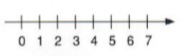We now wish to extend our thinking to include numbers to the left of zero. Such numbers are called negative numbers and are written with a minus sign preceding them. Numbers such as -6, -1, and -10 are read as "negative six," "negative one," and "negative ten."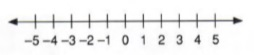The arrows indicate that the line continues indefinitely in either direction.

The whole numbers that we have studied up to this point and the negative whole numbers together make up the set of numbers called integers.

The set of numbers that can be expressed as the ratio of two integers is called the set of rational numbers. Fractions, such as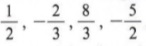will fall proportionally on the number line. The number line containing the rational numbers is called the rational number line.The numbers to the right of zero are called positive numbers and are written with a plus sign. If a nonzero number is written without a sign, it is assumed to be a positive number. Hence +7 or simply 7 would represent positive seven. Also note that "to the right" is a positive direction and "to the left" is a negative direction. Zero is neither positive nor negative.

These positive and negative numbers are referred to as signed numbers or directed numbers.

 What is the only unsigned number?

Most likely you have encountered signed numbers many times in your newspaper or on TV. For instance, if the temperature is ten degrees below zero, it is usually written as -10 degrees. If the stock market falls four points, it is usually written as - 4. You can probably think of other examples you have encountered.

Example 1 If a football team gained three yards on a play, how would you represent this using a signed number? How would you represent a loss of five yards?

Solution

+3 would represent a gain of three yards.
- 5 would represent a loss of five yards.

Example 2 Jack had a bank balance of \$23 and wrote a check for \$30. Represent his balance with a signed number.

Solution

Since he spent seven dollars more than he had, his balance is \$ - 7 and the banker is probably not too happy.

To be successful in using operations on signed numbers, a very important distinction must be made between a negative number and the negative of a number. We have already noted that a negative number is a number preceded by a minus sign. On the number line we noted that negative numbers are to the left of zero.

The negative of a number is the opposite of that number or that number with the opposite sign.

 A little lesson in English grammar: In "The negative of a number," negative is used as a noun. In "A negative number," negative is used as an adjective.

Example 3 The negative of +5 is -5.

Example 4 The negative of -5 is +5.

Example 5 The negative of +x is - x.

Example 6 The negative of - x is +x.

Example 7 If +7 represents 7 steps north, then -7 represents 7 steps south.

 We will agree that the negative of zero is zero.

Example 8 If -3 degrees represents three degrees below zero, then +3 degrees represents three degrees above zero.

Example 9 What is the negative of ( - 10)?

Solution

Since "the negative of" means "opposite of," the negative of -10 is +10.

The minus sign is often used as a symbol for "the negative of" or "opposite of." Therefore, -(-10) means "the opposite of" - 10.

 Note here two meanings of a minus sign.

Example 10 - (+7) = - 7

Example 11 -(-2) = +2

The distance from any number to zero on the number line is defined to be the absolute value of that number.

The absolute value of a number x is written as |x|, where
|x| = x if x is zero or positive
|x| = -x if x is negative

Example 12 |3| = 3

Example 13 | - 3| = 3

Notice that the absolute value of a number is always positive or zero.

In chapter 1 you learned that parentheses are used as grouping symbols. If we enclose a number expression in parentheses and place a minus sign before it, we are indicating the "negative" of the entire expression within the parentheses.

 Numbers or symbols grouped by parentheses are always regarded as a single number or symbol.

Example 14 - (a + b) means "the negative of the sum of a and b."

Example 15 - (x + 3 - b) means "the negative of, x plus 3 minus b."

To find the negative of an expression enclosed in grouping symbols find the negative of each term within the symbols.

Example 16 - (a + b) = - a - b

Example 17 -(x + 3 - b) = -x - 3 + b

Example 18 - (a - b) = - a + b

Example 19 -(a - 3 + 4 - x) = - a + 3 - 4 + x

 A very common error in finding the negative of an expression is not changing every sign. Be sure to express the opposite of every term within the grouping symbol if it is preceded by a minus sign.

Let us now restate the rule in more general terms.

To remove grouping symbols preceded by a minus sign change the sign of every term that was within the symbols. To remove grouping symbols preceded by a plus sign do not change the sign of any term that was within the symbols.

Example 20 - (a + 6 - 3) = -a - b + 3

Example 21 + (a + 6 - 3) = a + b - 3

Example 22 (x + y - a + b) = x+ y - a + b

## COMBINING SIGNED NUMBERS

### OBJECTIVES

Upon completing this section you should be able to:

1. Determine the result of combining two or more signed numbers.
2. Use the number line to combine signed numbers.

Example 1 If you start at a point and move six steps north then move three more steps north, where will you be in relation to your starting point?

Solution

The answer to this problem is nine steps north. If "steps north" is represented as positive, then six steps north followed by three steps north equals nine steps north could be written as +6 + 3 = +9.

Example 2 The temperature at 6:00 p.m. is zero degrees. From 6:00 p.m. until midnight the temperature falls three degrees, and from midnight until 4:00 a.m. it falls seven more degrees. What is the temperature at 4:00 a.m.?

Solution

If a fall in temperature is represented by a minus sign, then a fall of 3 degrees followed by a fall of 7 degrees can be written as

-3 degrees - 7 degrees = -10 degrees.

Example 3 At 9:00 a.m. the temperature is +20 degrees and from 9:00 a.m. until 1:00 p.m. it rises 14 degrees. Then from 1:00 p.m. until 5:00 p.m. it falls 18 degrees. What is the temperature at 5:00 p.m.?

Solution

Starting at +20 degrees, a rise of 14 degrees takes the temperature to +34 degrees. A fall of 18 degrees then takes it to +16 degrees. If a rise in temperature is positive and a fall in temperature is negative, then we can write

+ 20 degrees + 14 degrees - 18 degrees = +16 degrees.

The number line is a useful tool in combining signed numbers. If a movement "to the right" is considered + and a movement "to the left" is considered -, then consider the following examples.

 Unless the problem states otherwise, always start at zero for the first move.

Example 4 + 3 + 4 = +7 indicates "start at zero, move three units to the right, then move four units to the right." The result is a movement of seven units to the right.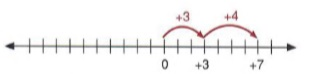Example 5 -2 - 4=-6 indicates "start at zero, move two units to the left, then move four units to the left." The result is a movement of six units to the left.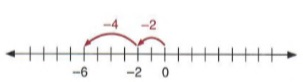Example 6 - 6 + 8 = +2 indicates "start at zero, move six units to the left, then move eight units to the right." The result is a movement of two units to the right.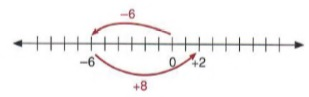Example 7 Combine: 3 - 6 + 4 - 5 =

Solution

Using the number line and starting at zero, first move three units to the right."+" is "to the right."

Then move six units to the left.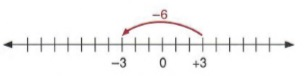Then move four units to the right.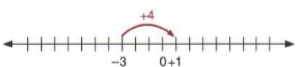"-" is "to the left."

Finally move five units to the left.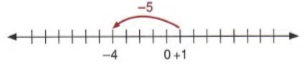The final location is at -4. Therefore, 3 - 6 + 4 - 5 = - 4.

 "-" is "to the left."

Example 8 After losing nine yards on the first down, the Browns completed a pass for a twelve-yard gain on the second down. What was the total gain or loss?

Solution

First write the number statement - 9 + 12 =
Using the number line, we obtain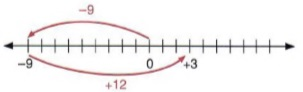Thus -9 + 12 = +3 (a three-yard gain).

## RULES FOR COMBINING SIGNED NUMBERS

### OBJECTIVES

Upon completing this section you should be able touse the rules for combining signed numbers.

In the preceding section we used the meaning of a signed number and the number line to work problems combining signed numbers. We are now ready to establish rules for performing this operation.

First we make the following observations from the discussion and examples of the preceding section.

1. A sign (plus or minus) affects only the number expression to its right.

Example 1 In +6 - 7 the + sign affects only the 6 and the - sign affects only the 7.

2. A sign preceding parentheses affects all terms inside the parentheses.

Example 2 In -( + 6 - 8) the - sign before the parentheses affects both the +6 and -8.

 Recall that if we remove parentheses, we would have -( + 6 - 8) = -6 + 8.

3. We combine numbers only two at a time.

Example 3 Combine: +6 + 4 + 3

Solution

We first combine +6 + 4 to obtain +10. Then we combine +10 + 3 to obtain +13.

 We could also first combine + 4 + 3 to get + 7, then combine + 6 + 7 toget + 13. This is due to the associative propoerty of addition that says (a + b) + c = a + (b + c)

Observation 3 makes it clear that we only need rules for combining two signed numbers.

To combine two numbers with the same sign add the numbers and attach the common sign.

Example 4 +6 + 8 = +14. We add 6 and 8 to obtain 14 and then attach the + sign that is common to both 6 and 8.

Example 5 - 6 - 8 = -14. We add the 6 and 8 to obtain 14 and then attach the - sign that is common to both 6 and 8.

To combine two numbers with unlike signs subtract the smaller number (without regard to sign) from the larger number (without regard to sign) and attach the sign of the larger number.

 If you move further to the right than to the left, you expect to end up to the right. Hence, use the sign of the larger number.

Example 6 - 7 + 11 = +4. We subtract 7 from 11 and use the + sign because 11 is larger than 7.

Example 7 +7 -11 = -4. We subtract 7 from 11 and use the - sign because 11 is the larger of the two numbers.

 Try working these examples using the number line to verify the answers.

Example 8 -14 + 8 = -6. We subtract 8 from 14 and use the - sign of 14.

Note again that rules for combining signed numbers apply to only two numbers at a time. If an expression contains several numbers, we must apply the rules more than once.

 Since only two numbers at a time may be added or subtracted, these operations are called binary operations.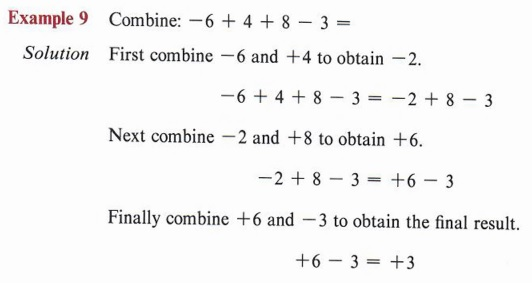Since the order of combining numbers will not change the answer (commutative property), we can, in example 9, proceed in a different way.Both of these approaches should be practiced so that you can choose whichever method is easier for a particular problem.

 In a problem such as this many students like to combine all the negative numbers together and all the positive numbers together then combine the two resulting numbers with unlike signs.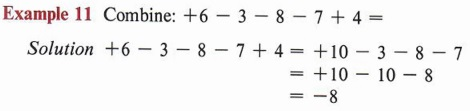Do the example by combining the numbers in order from left to right.

Note in this last example that this order gives easier combinations than combining left to right. The choice of order is left to the student, since the answer will be the same either way.

In section 1-2 we learned rules for removing grouping symbols. When combining numbers, we must be sure that each term involved has the proper sign. A term outside a grouping symbol should never be combined with a term inside the symbol, however, like terms within a grouping symbol should be combined before the symbol is removed.Remember that when removing grouping symbols preceded by a minus sign, change all signs. When removing grouping symbols preceded by a plus sign, do not change any sign.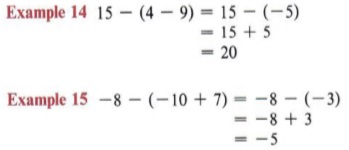Recall that when we simplify an expression containing grouping symbols within grouping symbols, we remove the innermost set of symbols first.Perform one operation at a time.

## COMBINING LIKE TERMS

### OBJECTIVES

Upon completing this section you should be able to:

1. Combine like terms involving signed numbers.
2. Remove grouping symbols and combine like terms.

In chapter 1 we defined like terms as terms that have exactly the same literal factors. We also noted that only like terms can be combined. We will now apply those same rules to terms involving signed numbers.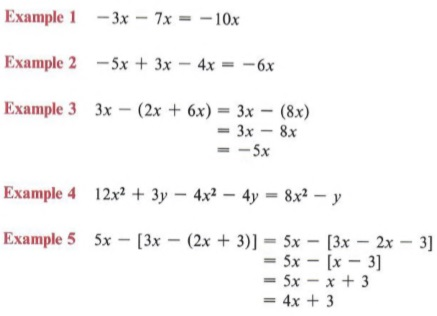### OBJECTIVES

Upon completing this section you should be able to:

1. Define subtraction in terms of addition.
2. Subtract signed numbers.

The symbol - is used to indicate both subtraction and the negative of. The expression 5x - ( - 4x) can be thought of as "subtract the negative of 4x from 5x" or as "add the negative of negative 4x to 5x." We have combined these like terms by thinking of this in the second way. We changed 5x - ( - 4x) to 5x + 4x and obtained 9x.

The following definition should make it clear that both statements are really the same.

Subtraction is adding the negative. In symbols
a - b = a + (-b).
(b subtracted from a is the same as the negative of b added to a.)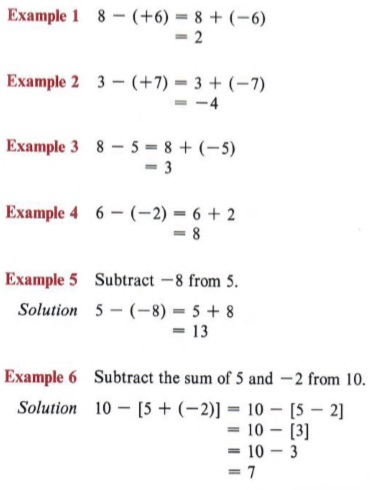Two signs are never written together without using grouping symbols.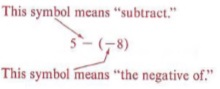Remember the order of removing grouping symbols.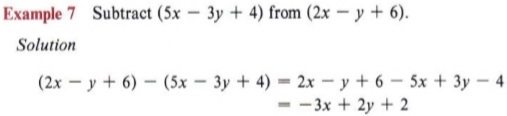## MULTIPLICATION AND DIVISION OF SIGNED NUMBERS

### OBJECTIVES

Upon completing this section you should be able to:

1. Apply the rule for multiplying signed numbers.
2. Apply the distributive property of multiplication over addition.
3. Divide signed numbers.

Multiplication can be thought of as "short-cut addition." For instance, if we wish to multiply (7)(5), we can think of this as "seven fives" or5 + 5 + 5 + 5 + 5 + 5 + 5 and obtain the result of 35. Or we could think of it as "five sevens" or 7 + 7 + 7 + 7 + 7 and also obtain the same result of 35.

Applying this same technique to signed numbers will lead to one of the rules for their multiplication.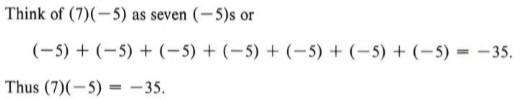The product of a positive number and a negative number yields a negative number.Remember that multiplying any number by zero always gives a product of zero. (5)(0) = 0 (-4)(0) = 0

From arithmetic we know the following rule.

The product of two positive numbers is positive.

In each of these rules be careful to note that the word "two" is very important. Since we can only multiply two numbers at a time, we only need rules for two numbers.

Example 4 Find the product: (3)(-2)(7)

Solution

There are several ways this could be done. We could first multiply (3)( - 2) obtaining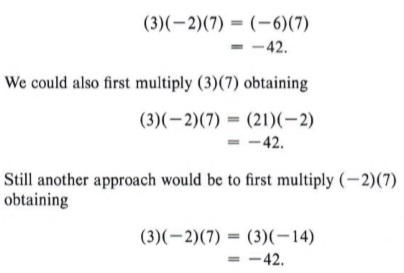It should be clear that the order in which the numbers are multiplied does not matter. The result will be the same.

 This is because multiplication is commutative. This is because multiplication is associative.That is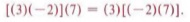To establish a rule for the product or quotient of two negative numbers we will need to use a property of the operations on numbers called the distributive property of multiplication over addition. In symbols the distributive property of multiplication over addition isNotice that a is a factor, that is, it is multiplying the quantity (b + c).

We may express this property in words by saying "to multiply terms enclosed in parentheses by a number we must multiply each term in the parentheses by that number."In an expression such as 2 + (x + 3) many students are tempted to multiply the expression in the parentheses by 2. But 2 is not a factor. The number directly multiplying the parentheses is (+1).If we use the distributive property, we have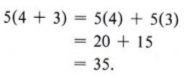Example 6 Remove parentheses and combine like terms:

Solution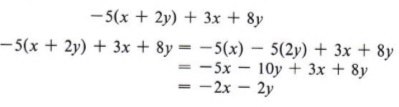We now wish to establish a rule for multiplying two negative numbers.

 When the sum of two numbers is zero, we say that one number is the additive inverse of the other. Since (5) + ( -5) = 0, we say 5 is the additive inverse of ( - 5).

By using our rules for adding signed numbers we have worked problems such as (-15) + (+15) = -15 + 15 = 0.

We also know that multiplying by zero gives zero as a result. For instance, ( -3)(0) = 0.

Now consider this problem. Find the result of ( - 3) [(5) + ( - 5)]. If we note that (5) + ( - 5) = 0, then we have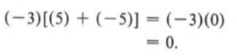However, if we first use the distributive property, we obtain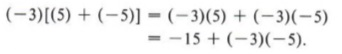Remember, the distributive property tells us to multiply each term inside the grouping symbols by the factor preceding the grouping symbols.

We know this result must be zero. That is, -15 + ( - 3)( - 5) = 0. Therefore, ( -3)( -5) = +15 since +15 is the only number that can be added to -15 to obtain a result of zero.

The choice of numbers in this discussion would not change the conclusion. Hence we have the following rule:

The product of two negative numbers is positive.Adding two negative numbers gives a negative number. Multiplying two negative numbers gives a positive number. Don't confuse these two rules.

Once more we must realize the importance of the word "two." This rule, as all others, must be applied to only two numbers at a time.

Example 10 Find the product of (- 3)(-2)( - 5).

Solution

If we first multiply (- 3)( - 2), we obtain ( + 6). Then multiplying ( + 6)( - 5), we obtain the final result.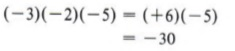Find this product by first multiplying (-2)(-5)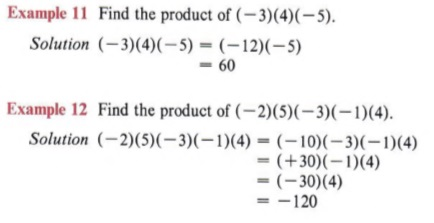Find this product by first multiplying (-3)(-5)

Division is defined as "multiplying by the inverse." The multiplicative inverse of a number is sometimes referred to as the reciprocal of the number.

In a problem such as 12 divided by 6, we are dividing by 6. Using the definition, the problem is the same as multiplying by the multiplicative inverse (or reciprocal) of 6, which is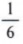.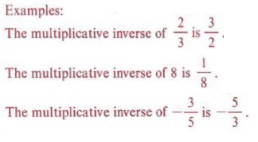This relationship ties division and multiplication together so that the rules for signs in division are the same as the rules for signs in multiplication. For convenience we will restate the rules as a single rule.

In multiplying or dividing signed numbers the product or quotient of two numbers with like signs is positive and the product or quotient of two numbers with unlike signs is negative.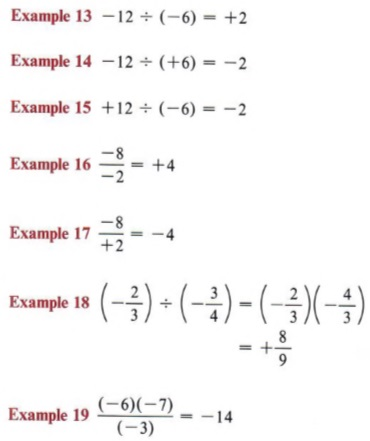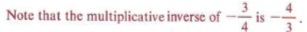## EXERCISES USING SIGNED NUMBERS

### OBJECTIVES

Upon completing this section you should be able to:

1. Simplify expressions involving signed numbers.
2. Evaluate expressions involving signed numbers.

We have used grouping symbols in several situations in the preceding chapters. Now that we have discussed the distributive property and the operations on signed numbers, we can extend our ability to remove grouping symbols.

 Remember to simplify the innermost grouping symbols first.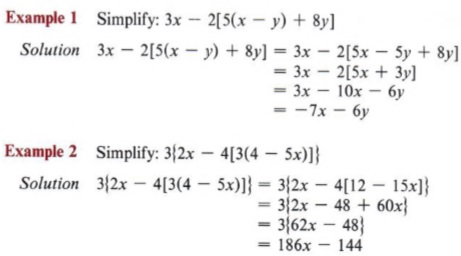In chapter 1 we evaluated literal expressions using only the numbers of arithmetic. We now are able to evaluate expressions that involve signed numbers.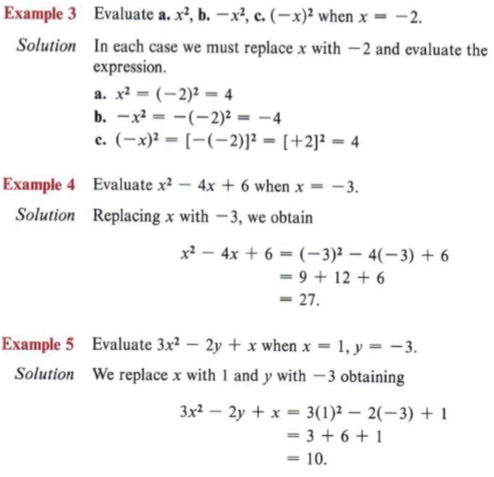Note the distinction between b and c. Remember that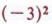means (- 3)(-3).

## SUMMARY

### Key Words

• A number line is useful for visualizing the relative positions of numbers.
• Signed numbers are preceded by a plus or minus sign.
• Positive numbers are numbers preceded by a plus sign.
• Negative numbers are numbers preceded by a minus sign.
• The negative of a number is that number with the opposite sign.
• The set of integers is composed of the whole numbers and the negative whole numbers.
• Rational numbers are those that can be expressed as the ratio of two integers.
• The absolute value of a number x is written as |x|, where
|x| = x if x is zero or positive.
|x| = -x if x is negative
• Combining numbers involves the operations of addition and subtraction.
• Subtraction is adding the opposite.
• The distributive property of multiplication over addition is a{b + c) = ab + ac.

### Procedures

• To find the negative of a number change its sign.
• To remove grouping symbols preceded by a minus sign find the negative of each term within the grouping symbols.
• To remove grouping symbols preceded by a plus sign do not change the sign of any term within the grouping symbols.
• If a movement to the right is considered positive and a movement to the left is negative, the number line can be used to combine signed numbers.
• To combine two numbers with like signs add the numbers and use the common sign.
• To combine two numbers with unlike signs, subtract the smaller from the larger (without regard to sign) and attach the sign of the larger.
• To multiply or divide signed numbers two like signs give a positive product or quotient and two unlike signs give a negative product or quotient.